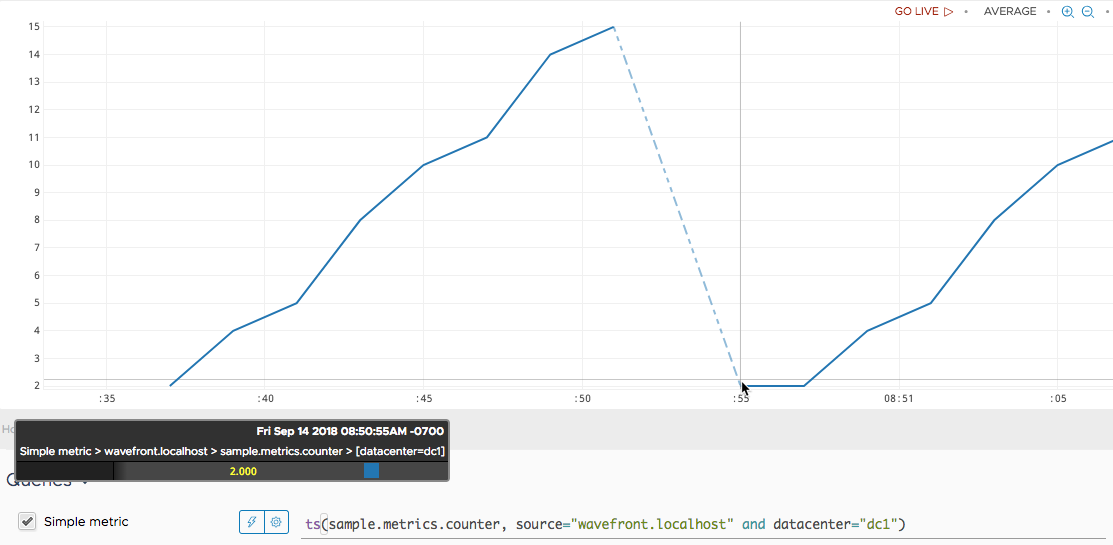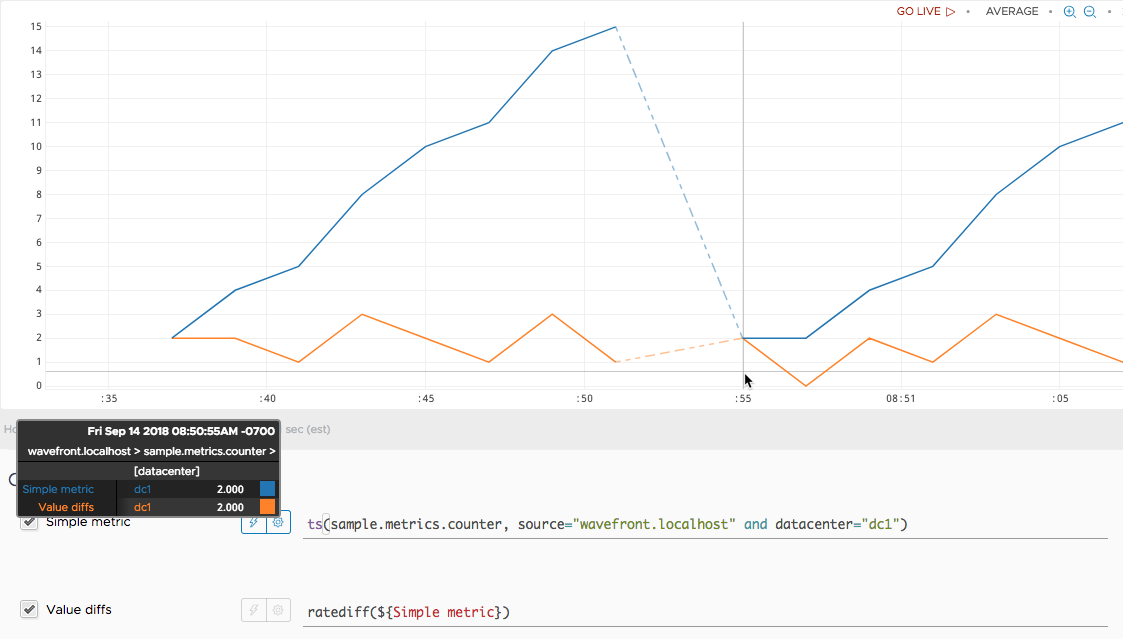Reference to the ratediff() function

## Summary

``````ratediff([<lookbackWindow>,] <tsExpression>)
``````

Returns the differences between adjacent values in each time series described by the expression. The results include only positive changes in value. Use `rate()` if you want to see per-second rates of change.

## Parameters

ParameterDescription
lookbackWindow Optional time window that specifies how far back an alert should look for the last known value.
tsExpression Expression describing the time series to return differences for.

## Description

The `ratediff()` standard time function returns the differences between adjacent increasing data values in the time series described by the expression. In contrast to `rate()`, this function returns the absolute difference between incrementing data points without dividing by the number of seconds between them. Here:

• `ratediff()` only gives you the difference in value between each two data points.
• `rate()` gives you the difference in value between each two data points divided by the number of seconds between them to give you the per/second rate.

For example, consider a metric that counts the cumulative number of logins for an application over time. You can use the `ratediff()` function to see how many logins were added from one reported data point to the next.

`ratediff()` returns a separate series of results for each time series described by the expression.

`ratediff()` returns only positive changes in value. As a result, it’s primarily useful for counter metrics, which are metrics that report cumulative totals (increasing values) over time.

`ratediff()` is similar to Graphite’s `nonNegativeDerivative()` function.

### Change in Value

`ratediff()` returns the change in value from one data point to the next, rather than computing a rate of change over time. For example, let’s say that a metric has a reporting interval of 30 seconds, and reports these successive data values:

 Value Time 105,500 05:45:00pm 105,750 05:45:30pm

`ratediff()` subtracts the second data value from the first one (`105,750 - 105,500`) and returns the resulting value (`250`) at 05:45:30pm. The difference between these data values would be exactly the same if the metric had reported them two minutes apart.

Because `ratediff()` ignores the time interval between reported data values, it is useful for time series that have gaps or irregular reporting intervals. Use `rate()` if you want to see per-second rates of change for time series with relatively regular reporting intervals and few gaps.

Note: `ratediff()` treats the first data value reported by a time series as if it were preceded by 0. Consequently, the first value returned by `ratediff()` is the same as the first data value in the input series.

### Response to Counter Resets

A counter metric normally produces an monotonically increasing series of data values, which produce positive changes in value. However, a metric might reset its counter on occasion – for example, if the metric’s source restarts.

A metric indicates a counter reset by reporting a lower data value immediately after a higher data value. `ratediff()` responds to a counter reset by returning the lower data value. Specifically, `ratediff()` treats the lower data value as if it were preceded by 0, and returns the difference between that value and the inferred 0.

`ratediff()` never returns a negative change in value.

### Improve Alert Accuracy with lookbackWindow Parameter

The optional first parameter is for situations where an alert does not check far back enough for the last known value and might behave incorrectly. Specify this parameter with the same units as a time window.

## Examples

Here’s a query that shows a sample metric that increments a counter. The reporting interval is 2 seconds, which means the counter increments every 2 seconds. We see the count climb from 8:50:36 to 8:50:51, when it resets. The counter restarts at 8:50:55.Now we apply `ratediff()` to the query find out how fast the counter grows from point to point.Notice:

• The first value returned by `ratediff()` is 2, which is the counter’s first value minus an inferred value of 0.
• `ratediff()` returns no value when the counter skips a point at roughly 8:50:53.
• When the counter restarts at 8:50:55, `ratediff()` returns 2, which is the counter’s first value after the restart, minus an inferred value of 0.
• The two points reported after the counter restarts have the same value (2), causing `ratediff()` to dip down to 0 at 8:50:57.